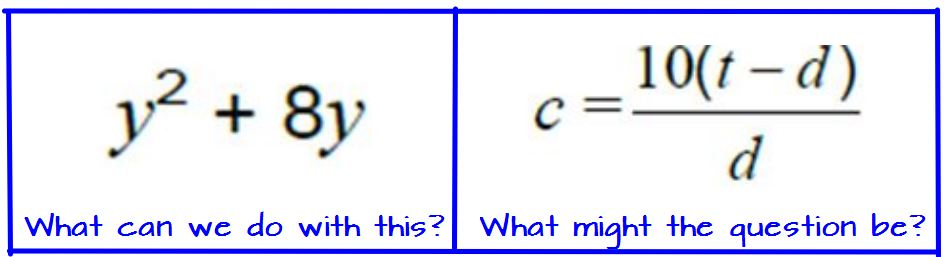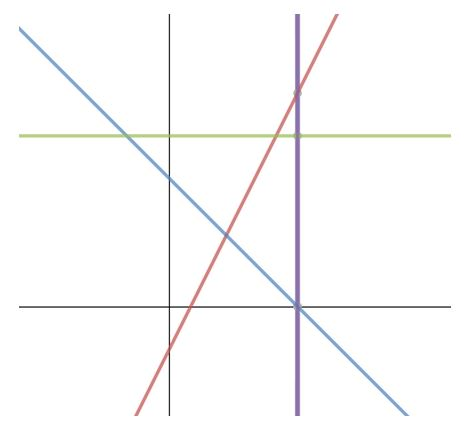# Algebra SnippetsYear 11 (UK age 15-16) have mock examinations starting next week so we have been revising.
I have written before on ‘Here’s the diagram …what’s the question? and having studied Trigonometry recently

the selection of various triangle diagrams worked very well and prompted some good discussion on unknowns we could work out in the various diagrams and what methods we could use.I thought I would extend the idea to Algebra and rather than a diagram just use what I have termed Algebra snippets where I have given for example only algebraic expressions, equations and so on from questions. (Very economical – you can get a lot on an A4 page!) All the vocabulary (which students often ignore!) has been stripped out. So rather like the diagrams idea students have to work out what the question might have been. With the vocabulary missing it is interesting to ask students what terms they think were used in the question. Following our discussions I also showed the students the complete questions. On the subject of vocabulary there are some excellent resources on this page including the very useful guide to exam terminology (I have seen this document around the web – I believe the original is from AQA).

Resources like the above can be fast to create – I created a table in Word then used the wonderful Windows Snipping tool to select my images and copy them to the table. If anyone wants the complete sheet: Word Algebra snippets or pdf Algebra snippets (you’ll see I snuck in a backwards percentage question as well).

I find these are worthwhile exercises and do get students thinking about the various question types and was pleased that some students started commenting on the differences in types of questions.One last diagram! Having asked these students if they wanted any particular topics, some asked for equations of lines so in one lesson we started with this diagram and managed to revise equations of lines, simultaneous equations and inequalities as well!

I gave them a copy of the diagram each and used a flipchart to give them some information and ask questions as we went along.

In case this is of interest the complete document is here: Lines & equations revision

This slideshow requires JavaScript.

The diagrams here were very easily created with Desmos, note that you can turn of numbers and/or the grid if you wish on Desmos from the Tools menu.